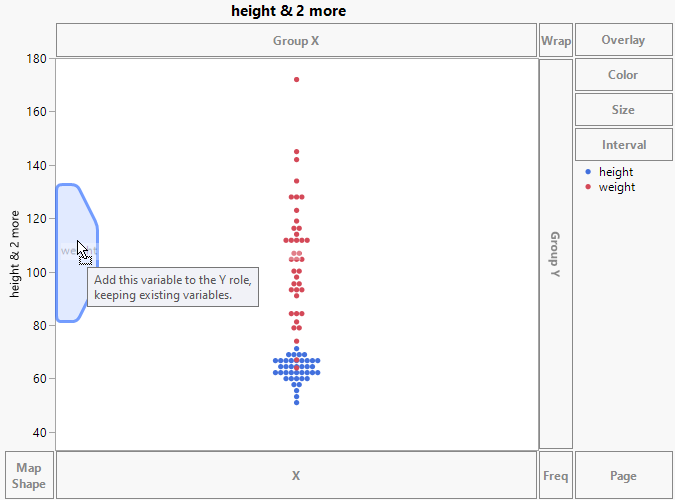Essential Graphing > Graph Builder > Work with Axes > Merge Variables on a Common Axis
Publication date: 05/24/2021

## Merge Variables on a Common Axis

Merging variables places both variables on the same axis and creates a single graph for both variables. Elements in the plot are colored by the variables, and a legend appears to the right of the plot. When points are plotted, they are randomly jittered for each value or category defined by the combination of the axes.

If you are starting with an empty zone, highlight multiple variables from the list and drag them into the zone at the same time.

If you already have variables in the zone and want to add more, drag the new variables to the inner side of the zone, in the graph area, until a symmetric polygonal shape appears.

Figure 3.11 New Variable Merged with Existing Variable#### Results Based on Data and Modeling Type

If only variables with continuous modeling types are merged, their values are plotted against a common axis.

If variables with categorical (nominal or ordinal) modeling types are merged with a continuous variable, the values of all variables are plotted against a common axis.

Before you can merge a variable with the character data type with numeric variables, the levels of the character variable have to be assigned numeric values. Integer values are assigned based on the Value Order column property or the default value ordering. These integer values range from 0 (highest level in value ordering) to the number of levels minus one (lowest value in value ordering). These integer values are then plotted against the common axis.

If only variables with categorical modeling types are merged, then separate, nested, axes are constructed for each variable. See Create Nested Axes for Character Variables.